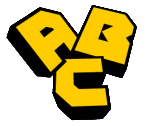### ABC-triplesOn this page you can find several lists of ABC triples:

The terminology is as follows. Suppose we have a solution in coprime positive integers of the equation a+b=c with a<b.

The radical r is the product of the distinct prime divisors of abc.
The quality is q=(log c)/log r.
The size is (log c)/log 10: the number of decimal digits of c.
The merit is defined to be (q-1)2(log r) log log r.

We say that the triple (a,b,c) is an ABC triple if r<c or, equivalently, if q>1. We say that one triple beats another if it has both a larger size and a larger quality.

The ABC conjecture says that the limsup of the quality when we range over all ABC triples, is 1. The unbeaten list provides the best known lower bound on how quickly this limsup tends to 1. The refined ABC conjecture of Robert, Stewart and Tenenbaum predicts the precise rate of convergence. It says that the limsup of the merit when we range over all ABC triples, is 48.

Links: